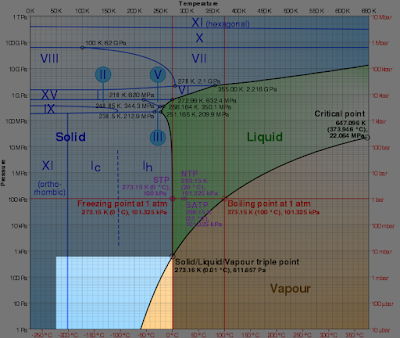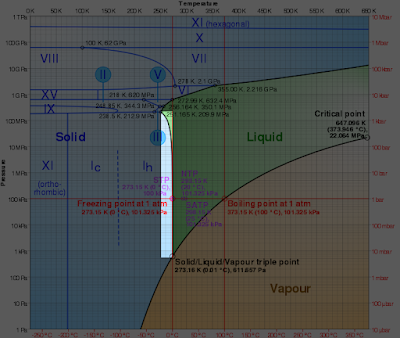## Featured Post

### The Need

Being able to calculate the state of water is somewhat important for the complete automation of the freeze dryer.  This will allow the computer to determine when the product has completely dried.  While the original plan was to watch the temperature and pressure to determine when to manually power off the freeze dryer, the RPi is perfectly capable of doing the work for us provided we can calculate when the product is dry.

As stated earlier, we know the state of water from the state-transition diagram.  When the state is near a line, we can assume both states bound by the line are present.  Likewise, when the temperature and pressure are not near a line, we can be sure only one state of water is present.

###This equation determines the water state in the highlighted region above.  Everything to the left of this region (lower temperature) is ice.  Everything to the right of this region (higher temperature) is vapor.

It is important to note in these calculations that pi is NOT pi in the traditional sense.  Instead of being 3.14159, pi is used as a variable in these equations.

We can calculate the sublimation/deposition line with a formula.  Without getting into the gory details, the sublimation line is curve-matched as follows:

ln(pi)=1/theta + sum_(i=1)^3 a_itheta^(b_i)

Where:
P_s= The pressure at the sublimation line in pascals
P_t= The pressure at the  L-G-S(Ih) triple point in pascals (611.657 Pa)
T_s= The temperature at the sublimation line in degrees kelvin
T_t= The temperature at the L-G-S(Ih) triple point in degrees kelvin (273.16 °K)

273.16 °K >=T_s>=50 °K

pi=P_s/P_t

theta=T_s/T_t

a_i{(-21.214\ 400 \ 6),(+27.320\ 381\ 9),(-\ \ 6.105\ 981\ 30):}

b_i{(+0.003\ 333\ 333\ 33),(+1.206\ 666\ 67),(+1.703\ 333\ 33):}

Through substitution we get:

ln(P_s/P_t)=1/((T_s/T_t))+sum_(i=1)^3 a_i (T_s/T_t)^(b_i)

ln(P_s/P_t)=T_t/T_s+sum_(i=1)^3 a_i (T_s/T_t)^(b_i)

P_s/P_t=e^([T_t/T_s+sum_(i=1)^3 a_i (T_s/T_t)^(b_i)])

P_s=P_t*e^([T_t/T_s+sum_(i=1)^3 a_i (T_s/T_t)^(b_i)])

P_s=P_t*e^([T_t/T_s + a_1 (T_s/T_t)^(b_1) + a_2 (T_s/T_t)^(b_2) + a_3 (T_s/T_t)^(b_3)])

P_s=P_t*e^([T_t/T_s + a_1 (T_s/T_t)^(b_1) + a_2 (T_s/T_t)^(b_2) + a_3 (T_s/T_t)^(b_3)])

With this equation, we can find the sublimation pressure P_s for any sublimation Temperature T_s.  We can then determine where the measured pressure lies in relation to the sublimation line. This will allow us to determine, programmatically, if the water in the product chamber is solid, gas, or a mixture of both.

We need to keep in mind that this equation is for the sublimation line ONLY.  It only works for the transition line between solid and gas phases.  This line only exists at pressures and temperatures below the triple point. This equation is ONLY valid between temperatures of 50 °K and 273.16 °K.

In this equation, all Temperatures are measured in degrees kelvin (°K) and all pressures are absolute pressures measured in pascals (Pa)

####Likewise, we can calculate the freeze/melt line. This equation determines the water state in the highlighted region above.  Everything to the right of this region (lower temperature) is ice.  Everything to the left of this region (higher temperature) is vapor or liquid, depending on the pressure.
This, along with the sublimation line, allows us to calculate all relevant boundaries for the solid state of water.    The solid state of water is more complex than this equation, but not in the regions the freeze dryer will be operating. This equation is as follows:
pi=1+sum_(i=1)^3 a_i(1-theta^(b_i ) )
Where:
P_m=The pressure at the melting line in pascals
P_t\ =The pressure at the L-G-S(I_h )triple point (611.657 Pa)
T_m=The temperature at the melting line in degrees kelvin
T_t\ =The temperature at the L-G-S(I_h )  triple point (273.16°K)

251.165 °K≥T_m≥273.16 °K

pi=P_m/P_t

theta=T_m/T_t

a_i {(+1\ 195\ 393.37),(+\ \ \ \ \ 80\ 818.315\ 9),(+\ \ \ \ \ \ \ 3\ 338.268\ 60):}

b_i {(+\ \ \ \ 3.000\ 00),(+\ \ 25.750\ 0),(+103.750):}

Through Substitution, we get:

P_m/P_t =1+ sum_(i=1)^3 a_i [1-(T_m/T_t)^(b_i ) ]

P_m/P_t=1+a_1[1-(T_m/T_t )^(b_1 ) ]+a_2[1-(T_m/T_t )^(b_2 ) ]+a_3[1-(T_m/T_t )^(b_3 ) ]

P_m=P_t{1+a_1 [1-(T_m/T_t )^(b_1 ) ]+a_2 [1-(T_m/T_t )^(b_2 ) ]+a_3[1-(T_m/T_t )^(b_3 ) ]}

With this equation, we can find the melting pressure P_m for any temperature T_m.  We can then determine where the measured pressure lies in relation to the melting line. This will allow us to determine, programmatically, if the water in the product chamber is solid, liquid, or a mixture of both.

We need to keep in mind that this equation is for the melt line ONLY.  It only works for the transition line between solid and liquid phases.  This equation is ONLY valid between temperatures of 251.165 °K and 273.16 °K.

In this equation, all temperatures are measured in degrees kelvin (°K) and all pressures are absolute pressures measured in pascals (Pa)

The line plotted for this particular curve is quite steep and probably serves little purpose in the freeze dryer project for a couple of reasons.  First, this line is entirely above the triple point.  As mentioned before, the freeze-drying process takes place below the triple point.  Second, since this line is so steep, we are probably not going to need to ride this line too much.  All aspects of the freeze dryer temperature will be to the left of this line.  For the most part, this section was included for completeness.

#### Other Freeze/Melt Equations

There are other freeze/melt equations, but these phase changes take place at pressures far above the pressures a freeze dryer operates at and will not be covered here.  For more information read "New Equations for the Sublimation Pressure and Melting Pressure of H2O Ice Ih" by Wolfgang Wagnera, Thomas Riethmann, Rainer Feistel and Allan H. Harvey